# Oranges

Mother divided her three children's oranges in a ratio of 6:5:4. Two children gave 45 oranges. How many oranges were there?

n =  75

### Step-by-step explanation: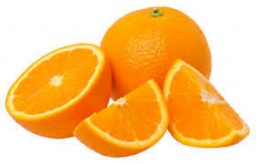Did you find an error or inaccuracy? Feel free to write us. Thank you!Tips to related online calculators
Need help to calculate sum, simplify or multiply fractions? Try our fraction calculator.
Check out our ratio calculator.
Do you have a linear equation or system of equations and looking for its solution? Or do you have a quadratic equation?

## Related math problems and questions:

• TogetherThe three friends divided the balls in a ratio of 6: 5: 4. Some two of them got a total of 126 balls. How many balls were there together?
• The ratio 4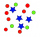The ratio of two number is 5:4 if 40% of the first number is 12, what will be 50% of the second number?
• GroupA group of kids wanted to ride. When the children were divided into groups of 3 children, one remain. When divided into groups of 4 children, 1 remains. When divided into groups of 6 children, 1 remains. After divided into groups of 5 children, no one lef
• Apples 5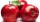In six crates are 45 kg of apples. In five crates were the same amount and in one crate was 3 kg of apples more. How many kg of apples were in each crate?
• TogetherIf 8 men, 10 women, 16 children collects ₹1024 in 4 days, how many days will be required for 6 men, 5 women and 4 boys to collect ₹768? (₹ is Indian Rupee)
• Hotel roomsIn the 45 rooms, there were 169 guests. Some rooms were three-bedrooms and some five-bedrooms. How many rooms were there?
• Boys and girls 8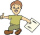In a class of 35 boys and girls that has a ratio of 2:5, 5 boys are absent and 5 boys are present. How many students are present?
• Trio ratioHans, Alena, and Thomas have a total of 740 USD. Hans and Alena split in the ratio 5: 6 and Alena and Thomas in the ratio 4: 5. How much will everyone get?
• 45th birthdayThis year Mrs. Clever celebrated her 45th birthday. Her three children are now 7, 11 and 15 years old. In how many years will Mrs. Clever's age be equal to the sum of her children's years?
• Mother and daughterThe mother is four times older than her daughter. Five years ago, her daughter was seven times younger than her mother. How many years do they have now?
• Pears and orangesThere are 3 times as many pears as oranges. If a group of children receives 5 oranges each, there will be no oranges left over. If the same group of children receives 8 pears each, there will be 21 pears leftover. How many children and oranges are there?
• Sheep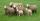Shepherd tending the sheep. Tourists asked him how much they have. The shepherd said, "there are fewer than 500. If I them lined up in 4-row 3 remain. If in 5-row 4 remain. If in 6-row 5 remain. But I can form 7-row." How many sheep have herdsman?
• Z9–I–4 MO 2017Numbers 1, 2, 3, 4, 5, 6, 7, 8 and 9 were prepared for a train journey with three wagons. They wanted to sit out so that three numbers were seated in each carriage and the largest of each of the three was equal to the sum of the remaining two. The conduct
• Large familyThe average age of all family members (children, mother, father, grandmother, grandfather) is 29 years. The average age of parents is 40 years, grandparents 66 years and all children are 5 years. How many children are there in this family?
• Blueberries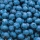5 children collect 4 liters of blueberries in 1.5 hours. a) How many minutes do 3 children take 2 liters of blueberries? b) How many liters of blueberries will be taken by 8 children in 3 hours?
• Five numbers in ratioFive integers are in the ratio 1: 2: 3: 4: 5. Their arithmetic mean is 12. Determine the smallest of these numbers.
• There 5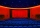There were 429 people at the play. Admission was 50 pesos each for adults and 35 pesos each for children. The receipts were 17265 pesos. How many adults and how many children attended?